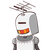# Mr. Sharma lent 15000 to his friend. He charged the interest at 15% per annum on12500 and 18% on the rest. How much interest

Mr. Sharma lent 15000 to his friend. He charged the interest at 15% per annum on
12500 and 18% on the rest. How much interest did he earn in 3 years ?​

### 1 thought on “Mr. Sharma lent 15000 to his friend. He charged the interest at 15% per annum on<br />12500 and 18% on the rest. How much interest”

1.### Given:–

• Principal (p) = Rs. 12500
• Time (T) = 3 years
• Rate of interest (R) = 15% per annum

### Tofind:–

• The interest

we know,

$$\bf \pink{Interest= \frac{p \times r \times t \: }{100} }$$

Where,

P is the principal, r is the rate of interest and t is the time period.

Now, By putting the values we get,

$$\implies\sf{ \frac{12500 \times 15 \times 3}{100} }$$

$$\sf \implies \: 5625$$

Therefore, the interest in this case is Rs 5625 .

Rest amount we get as,

$$\sf \implies \: 15000 – 12500 = 2500$$

We know, the rate of interest on this is 18%

So,

The interest on Rs. 2500 for 3 years we get,

$$\sf\implies{Interest = \frac{p \times r \times t}{100} }$$

Now,

By putting the value,

$$\sf\implies{ \frac{2500 \times 18 \times 3}{100} }$$

$$\sf\implies{1350}$$

So,

The total interest earned in 3 years

is

$$\sf\implies{5625 + 1350 = 6975}$$

Hence,

$$\small\bf\purple{Hope\:it\:helps\:uh\::)}$$